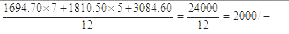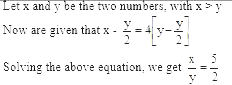# Sample Aptitude Questions of Mahindra & Mahindra

1. A cricketer played 80 innings and scored an average of 99 runs. His score in the last inning was zero run. To have an average of 100 at the end, his score in the last innings should have been
1. 60
2. 80
3. 10
4. 1
5. None of these

Let x be the score in the last inning to make the average of 100
Therefore, when average is 99, total score of 80 innings = 80 * 99 = 7920
And when the average is 100, total score of 80 innings = 80 * 100 = 8000
Therefore, x = 8000 - 7920 = 80
2. A man spends an average of Rs. 1,694.70 per month for the first 7 months and Rs.1,810.50 per month for the next 5 months. His monthly salary if he saves Rs. 3,084.60 during the whole year is
1. 1000
2. 2000
3. 2400
4. 3000
5. None of these

Monthly salary =1. A and B undertake to do a piece of work for Rs. 2,200. A alone can do it in 8 days, while B can do it in 6 days. With the help of C, they complete it in 3 days. Find C's share.
1. 150
2. 275
3. 245
4. 175
5. None of these

Let 1 be the total work
Therefore, C's one day work = (1/3)-[(1/8)+(1/6)]= 1/24
Ratio of share of A, B and C
i.e. A:B:C = 1/8:1/6:1/24=> [1/3] - {[1/8]+[1/6]} = (1/24) 3 : 4 : 1
So, C's share = {1/8} *2200=275-/
2. By selling an article at 80% of its marked price, a trader makes a loss of 10%. What will be the profit percentage if he sells it at 95% of its marked price?
1. 5.9
2. 12.5
3. 6.9
4. 5
5. None of these

Let us assume the MP to be 900/-
Therefore, SP = 80% of MP = 0.80 * 900 = 720/-
As loss is 10%, so CP = 720/90 *100=800
Now, if SP = 95% of MP = 0.95 *900 = 855/-
Profit% = 855-800 /800 =55/8=6.9
3. By selling an umbrella for Rs. 30, a shopkeeper gains 20%. During a clearance sale, the shopkeeper allows a discount of 10% of the marked price. His gain during the sale season is
1. 8
2. 9
3. 7
4. 7.5
5. None of these

Given that SP of umbrella = 30/- and profit% = 20. Therefore, CP of umbrella = 30/120 *100=25 SP of umbrella after 10% discount = 90/100 *30=27 Thus, profit% = 27-25 /25 =8
4. From a vessel containing 100 ltr. of wine, 10 ltr. are drawn out and an equal amount of water is added. From the mixture, 10 ltr. is again drawn out and same quantity of water is added. What is the final ratio of wine and water?
1. 91:9
2. 81:19
3. 80:20
4. 90:10
5. None of these

This is the question of removal and replacement If a vessel contains "x" litres of liquid A and if "y" litres be withdrawn and replaced by liquid B, then if "y" litres of the mixture be withdrawn and replaced by liquid B again, and the operation is repeated "n" times in all, then:So, in the given question, we have A as wine and B as water and 2 operations have occurred.
Therefore, quantity of wine after 2 operations
Thus, in a mixture of 100 ltr, 81 is wine, so water will be 100 - 81 = 19 ltr
Required ratio of wine and water is 81 : 19
5. From each of two given numbers, half the smaller number is subtracted. After such subtraction, the larger number is 4 times as large as the smaller number. What is the ratio of the numbers?
1. 4:1
2. 4:2
3. 5:2
4. 1:4
5. None of these6. Men, women and children are employed to do a work in the proportion of 3 : 2 : 1 and their wages per person are in the proportion of 5 : 3 : 2. When 90 men are employed, total daily wages of all amounts to Rs. 10,350. Find the daily wage of a man.
1. 115
2. 75
3. 45
4. 57.90
5. None of these

Let the daily wages be 5k, 3k and 2k of men, women and children respectively.
We are given that there are 90 men and total amount of wages is 10350/-
Thus, men, women and children are 90, 60 and 30 respectively.
Hence, total wages = 90 x 5k + 60 x 3k + 30 x 2k = 10350
=>k = Rs.15
Thus, daily wages of each man = 5k = Rs.15 x 5 = Rs. 75
7. The population of a town is 3,11,250. The ratio between women and men is 43 : 40. If there are 24% literate among men and 8% literate among women, the total number of literate persons in the town is
1. 56,800
2. 99,600
3. 41,800
4. 48,900
5. None of these

Total number of literate people is 24 % of 40 % of men and 8 % of 43 % of women.
Therefore, No of literate persons = [24/100 *40/83* 311250 ] + [ 8/100 * 43/83 * 311250] = 48900
8. In an examination, 52% of the candidates failed in English and 43% failed in Mathematics. If 17% failed in both the subjects, then the percentage of candidates, who passed in both the subjects, was
1. 25
2. 22
3. 23
4. 21
5. None of The above